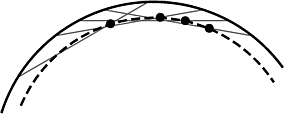## Maintaining the Extent of a Moving Point Set

Reference: Maintaining the Extent of a Moving Point Set, with Pankaj K. Agarwal, Leonidas J. Guibas, and John Hershberger, 5th Workshop on Algorithms and Data Structures (WADS '97), August 1997.

### Abstract

Let $S$ be a set of $n$ moving points in the plane. We give new efficient and compact kinetic data structures for maintaining the diameter, width, and smallest area or perimeter bounding rectangle of the points. When the points in $S$ move with pseudo-algebraic motions, these structures process $O(n^{2+\epsilon})$ events. We also give constructions showing that $\Omega(n^2)$ combinatorial changes are possible in these extent functions even when the points move on straight lines with constant velocities. We give a similar construction and upper bound for the convex hull, improving known results.

### Additional information

Return to other recent papers from Stanford

Last modified: January 22, 1998

Eric Veach